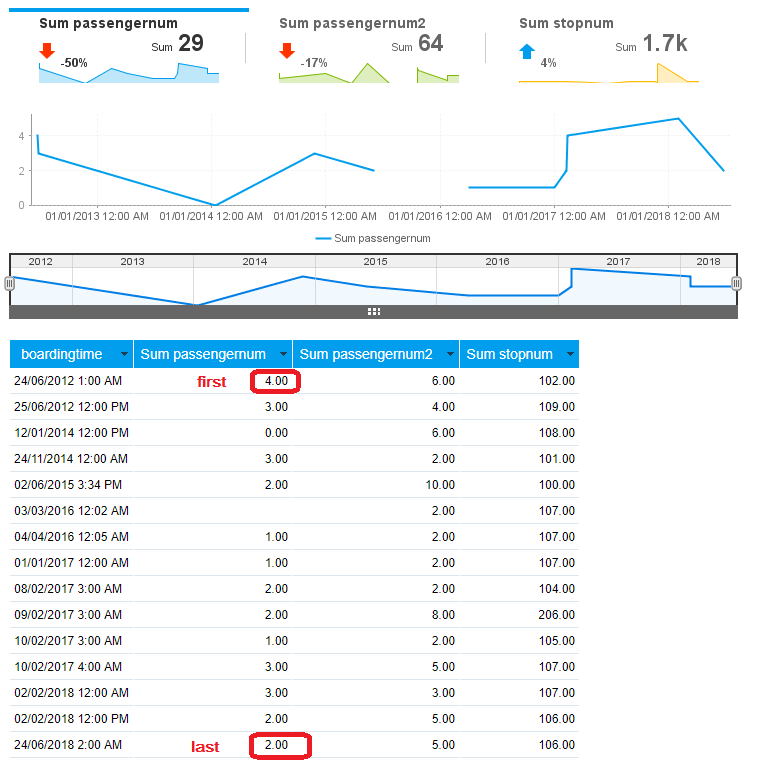# Percentage in visible series selection

Stephanus Adisuprijanto shared this question ago

Can someone explain it to me how Yellowfin calculate the percentage number displayed when we activate the visible series selection?

I notice that it also changes whenever date slider is updated by users.1

Hi Stephanus,

let x = first metric in time frame indicated by the date slider

let y = last metric in time frame indicated by the date slider

then the formula is as follows:

( ( y - x ) / x ) * 100

regards,

David1

Hi David,

Can you explain to me what you meant by first metric and last metric?

Is the value of the first day/week/month/quarter/year (whatever time unit we chose) the first metric and the value of the last day/week/month/quarter/year (whatever time unit we chose) the last metric?2

Hi Stephanus,

sorry, I should have said "value" instead.

Here is an example:thus for the Passengernum series it is:

( ( 2 - 4 ) / 4 ) * 100

= ( -2 / 4 ) * 100

= -0.5 * 100

= -50%

regards,

David1

OK got it thanks David.

Regards

Stephanus